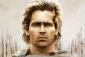# Minima maxima: Arbitrary constants for a cubicMake the curve y=ax³+bx²+cx+d pass through the points (0,1)and (-3,7) and have a critical point at (-1,3)

Ans. y=-x³-4x²-5x+1
Thanks po### Steps:

Steps:

1. Substitute (0, 1) to the equation.
Result: d = 1.
2. Substitute (-3, 7) to the equation.
Result: -27a + 9b - 3c = 6.
3. Substitute (-1, 3) to the equation.
Result: -a + b - c = 2.
4. Differentiate and set y' to zero. Because (-1, 3) is a critical point, replace x with -1.
Result: 3a - 2b + c = 0.
5. Solve the three equations from steps (2), (3), and (4).### Paano ko po makuha yung value

Paano ko po makuha yung value ni a at b?

Eqn.2 at eqn 3 nalang po natira.
0=0 po yung eqn.4
C=-3a+2b kasi value ni c. At sinubstitute ko sa eqn. 2-4### Eq. (1):   -27a + 9b - 3c = 6

Eq. (2):   -27a + 9b - 3c = 6
Eq. (3):   -a + b - c = 2
Eq. (4):   3a - 2b + c = 0

Eq. (2) - 3*Eq. (3)
-24a + 6b = 0
6b = 24a
b = 4a

Eq. (3) + Eq. (4)
2a - b = 2
2a - 4a = 2
-2a = 2
a = -1### Thank you sir Alexander and

Thank you sir Alexander and Sir Jhun Vert

• Mathematics inside the configured delimiters is rendered by MathJax. The default math delimiters are $$...$$ and $...$ for displayed mathematics, and $...$ and $...$ for in-line mathematics.